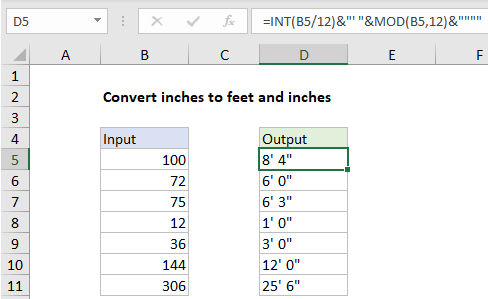# Convert inches to feet and inches in Excel

To convert a measurement in inches to inches and feet (i.e. 53 to 4′ 5″) you can use a formula based on the INT and MOD functions. See illustration below:

## Formula

`=INT(A1/12)&"' "&MOD(A1)&""""`## Explanation

In the example shown, the formula in D5 is:

`=INT(B5/12)&"' "&MOD(B5,12)&""""`

### How this formula works

This formula converts a numeric value in inches to text representing the same measurement in inches and feet. To get the value for feet, the INT function is used like this:

`=INT(B5/12)&"' "`

Inside INT, the value in B5 is divided by 12 and INT simply returns the integer portion of the result, discarding any decimal remainder. The result is then concatenated to a string with a single quote and space character.

Worked Example:   Extract date from a date and time in Excel

To get a value for inches, the MOD function is used like this:

`MOD(B5,12)&""""`

where number comes from B5 and the divisor is 12. Configured in this way, MOD returns the remainder after division. The result is concatenated to two sets of double quotes. The outer pair indicates text, and inner pair is needed for Excel to output a single double quote.

Worked Example:   Basic timesheet formula with breaks in Excel

Finally, the INT code and MOD code are concatenated together and Excel returns the final text value.

Worked Example:   Convert column letter to number in Excel

### With complete labels

To output a value like “8 feet 4 inches”, you adapt the formula like this:

`=INT(B5/12)&" feet "&MOD(B5,12)&" inches"`